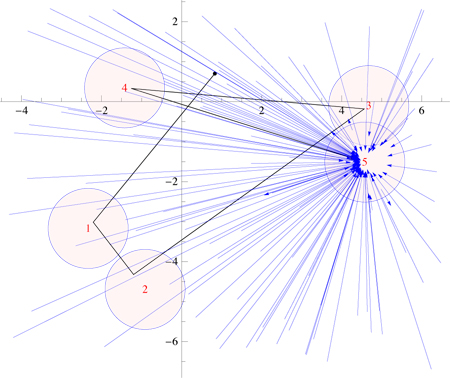# Composition of circle inversions

I would like to understand the map of $\mathbb{C}$ to $\mathbb{C}$ that results by iterating inversion in a unit circle. Let $f(z)$ for $z \in \mathbb{C}$ invert $z$ in a unit circle centered on $q_1$, and then in a unit circle centered on $q_2$, etc. Here is an example:The black path shows the trajectory of one point $z$, which ends up inside the $q_5$ disk. The blue vectors show the complete map's effect on a number of (random) points. The end result resembles an inversion in the $q_5$ circle, but I imagine the plane is partitioned into regions that behave similarly due to their relationship to the several circles of inversion. Is this a correct way to view this map? And if so, is there a natural diagram to elucidate the partition?

I wonder if instead it may be better to view this map as an approximation to another map? For there is a continued-fraction representation of $f(z)$, as follows. The inversion of $z$ in a unit circle centered on $q$ can be expressed as $q + \frac{1}{z^* -q^*}$, where ${\cdot}^*$ represents the complex conjugate operation. Then iterating this (say, five times) leads to this expression: $$f(z) = q_5 + \frac{1} {q_4^*-q_5^* + \frac{1} {q_3-q_4+ \frac{1} {q_2^*-q_3^*+ \frac{1} {q_1-q_2+\frac{1}{z^*-q_1^*} } } } }$$

I realize I have not posed a sharp question. I am just seeking some approach that helps understand the composition of circle inversions, a bit far from my expertise. Thanks for pointers or ideas!

• As far as I can see, the composition of $n$ inversions is a Möbius transformation, or a Möbius transformation followed by conjugation, depending on the parity of $n$. It thus should not involve any partitions. – Emil Jeřábek Dec 14 '11 at 15:42
• @Emil: Interesting! And yet different $z$s follow rather disparate paths depending on whether they land in or out of specific disks. Which is not to say I doubt you--rather, I appreciate this insight all the more! – Joseph O'Rourke Dec 14 '11 at 16:31
• This is just a bit of free association, but your question reminds me a bit of the notion of a Schottky group en.wikipedia.org/wiki/Schottky_group . I learned about them from the fantastic book "Indra's Pearls" by Mumford, Series, and Wright. – j.c. Dec 14 '11 at 18:23

In terms of complex number coordinates for the planes, the compositions of even numbers of inversions are the fractional linear transformations $$z\mapsto \frac{az+b}{cz+d}$$ (where $a$, $b$, $c$, and $d$ are complex numbers with $ad-bc\ne 0$, and multiplying all four of them by the same scalar leaves the transformation unchanged). The compositions of odd numbers of inversions are their complex conjugates.